# normalized data multi line chart

I have several variables ( impression, cost, return on investment ratio) and I would like to normalize them to range from 1 and -1. Is there any way I can do it? Thanks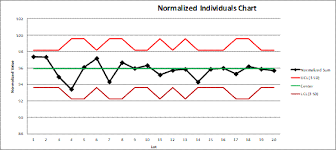• See if this works : )

Create a formula in beast mode :

case when `Account Name` = 'Cost' then (`Value` - min(`Value`) ) / (max(`Value`) - min(`Value`) ) end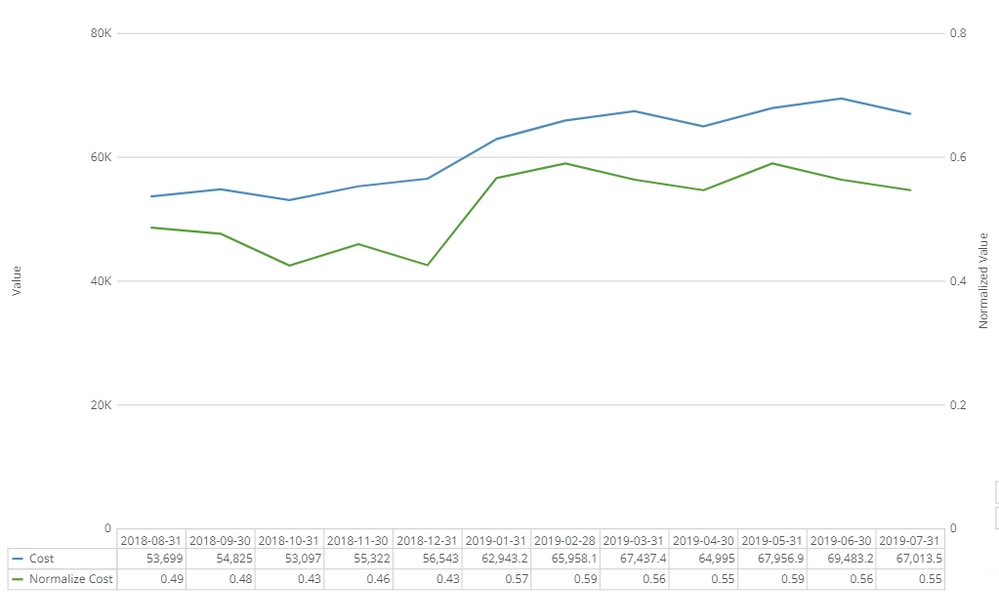• Hi, Thanks for your reply. I have tried it and it didn't work, it's validated but the graph won't appear. Can you explain to me why there's a different value trend on 30-11-2018 and 30-12-2018 where the normalized value should be uptrend?

• Ah sorry - I was using a different axis (my bad). Let me try again. Attached is a simple sample data set that I used (just focusing on CostA)

CostA" = (`CostA` - min(`CostA`) over (partition by year(`Date`))) /
(max(`CostA`) over (partition by year(`Date`)) - min(`CostA`) over (partition by year(`Date`)))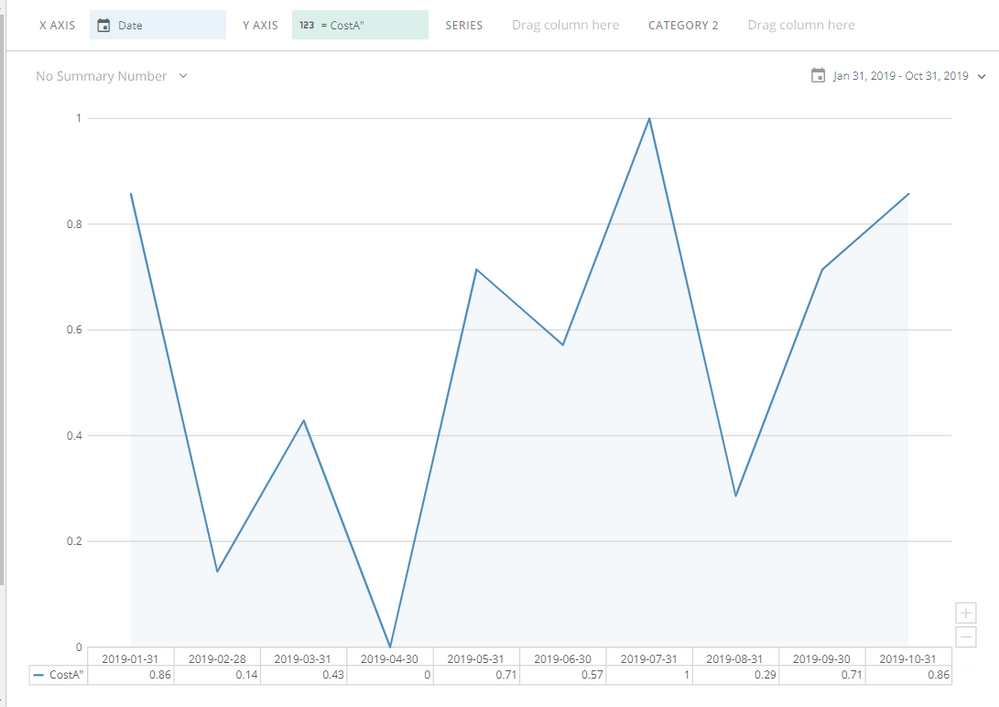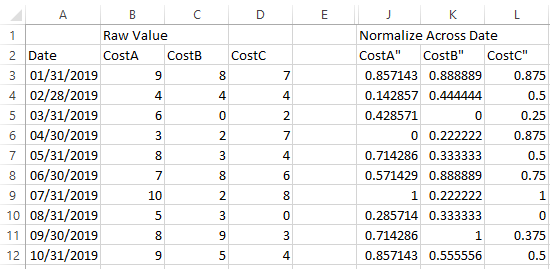• and to get between -1 and 1 I used :

2*
(`CostA` - min(`CostA`) over (partition by year(`Date`))) /
(max(`CostA`) over (partition by year(`Date`)) - min(`CostA`) over (partition by year(`Date`)))
-1

• It works well but if I have a duplicate date, the chart didn't appear.

Let's say if I have duplicate date data from my raw data what should I do?. I would like to see the overall normalized cost regarding the account the option of filter the Account without changing the raw data.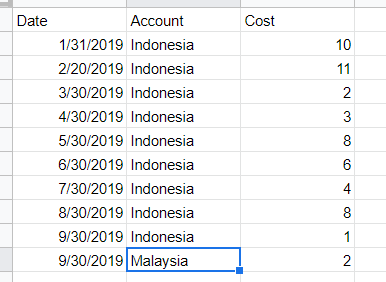• Heya - Had a play with this although with not much more success as I am not sure how the overall (column O) number should look like.  But to get the individual account, I added Account to the filter and changed the beast calc to:

2*
(`CostA` - min(`CostA`) over (partition by year(`Date`),`Account`)) /
(max(`CostA`) over (partition by year(`Date`),`Account`) - min(`CostA`) over (partition by year(`Date`),`Account`))
-1

This discussion has been closed.